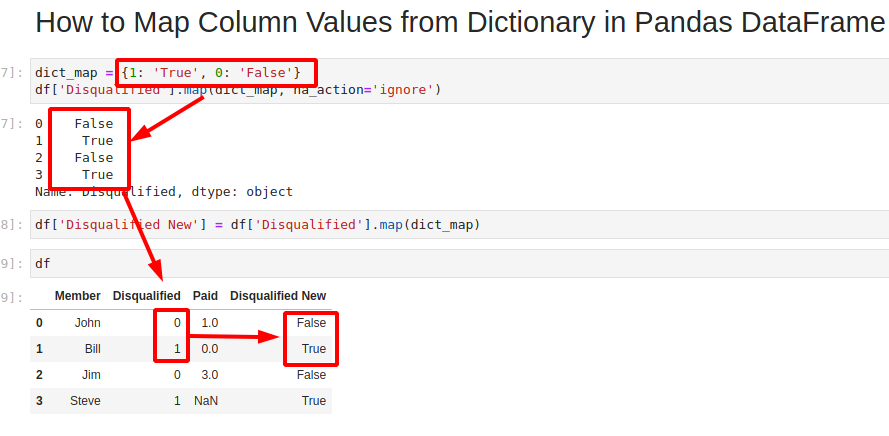## 1. Overview

In this tutorial, we'll learn how to map column with dictionary in Pandas DataFrame. We are going to use Pandas method pandas.Series.map which is described as:

Map values of Series according to an input mapping or function.

There are several different scenarios and considerations:

• remap values in the same column
• add new column with mapped values from another column
• keep existing values

Let's cover all examples in the next sections. The image below illustrates how to map column values work:## 2. Setup

In the post, we'll use the following DataFrame, which consists of several rows and columns:

``````import pandas as pd
import numpy as np

data = {'Member': {0: 'John', 1: 'Bill', 2: 'Jim', 3: 'Steve'},
'Disqualified': {0: 0, 1: 1, 2: 0, 3: 1},
'Paid': {0: 1, 1: 0, 2: 0, 3: np.nan}}

df = pd.DataFrame(data)
``````

Data looks like:

Member Disqualified Paid
0 John 0 1.0
1 Bill 1 0.0
2 Jim 0 0.0
3 Steve 1 NaN

## 3. Pandas map Column with Dictionary

First let's start with the most simple case - map values of column with dictionary.

We are going to use method - pandas.Series.map.

We are going to map column Disqualified to boolean values - 1 will be mapped as `True` and 0 will be mapped as `False`:

``````dict_map = {1: 'True', 0: 'False'}
df['Disqualified'].map(dict_map)
``````

The result is a new Pandas Series with the mapped values:

``````0    False
1     True
2    False
3     True
Name: Disqualified, dtype: object
``````

### 3.1 Map column values in DataFrame

We can assign this result Series to the same column by:

``````df['Disqualified'] = df['Disqualified'].map(dict_map)
``````

### 3.2 Map dictionary to new column in Pandas

To map dictionary from existing column to new column we need to change column name:

``````df['Disqualified Boolean'] = df['Disqualified'].map(dict_map)
``````
Note:

In case of a different DataFrame be sure that indices match

## 4. Mapping column values and preserve values(NaN)

What will happen if a value is not present in the mapping dictionary? In this case we will end with `NA` value:

``````df['Paid'].map(dict_map )
``````

result:

``````0     True
1    False
2      NaN
3      NaN
Name: Paid, dtype: object
``````

In order to keep the not mapped values in the result Series we need to fill all missing values with the values from the column:

``````df['Paid'].map(dict_map).fillna(df['Paid'])
``````

This will result into:

``````0     True
1    False
2      3.0
3      NaN
Name: Paid, dtype: object
``````

To keep NaNs we can add parameter - `na_action='ignore'`:

``````df['Disqualified'].map(dict_map, na_action='ignore')
``````

## 5. Map Column in Pandas - map() vs replace()

An alternative solution to map column to dict is by using the function pandas.Series.replace.

The syntax is similar but the result is a bit different:

``````df["Paid"].replace(dict_map)
``````

In the result Series the original values of the column will be present:

``````0     True
1    False
2      3.0
3      NaN
Name: Paid, dtype: object
``````

Another difference between functions map() and replace() are the parameters:

• `.replace(dict_map, inplace=True)` - applying changes on the Series itself
• `df['Paid'].map(dict_map, na_action='ignore') - to avoid applying the function to missing values (and keep them as NaN)

Finally we can mention that `replace()` can be much slower in some cases.

## 6. Map column with s.update() in Pandas

Another option to map values of a column based on a dictionary values is by using method `s.update()` - pandas.Series.update

This can be done by:

``````df['Paid'].update(pd.Series(dict_map))
``````

The result will be update on the existing values in the column:

``````0    False
1     True
2      3.0
3      NaN
Name: Paid, dtype: object
``````

The function is described as:

Modify Series in place using values from passed Series.
Uses non-NA values from passed Series to make updates. Aligns on index

## 7. Map dictionary to new column in Pandas DataFrame

Finally we can use pd.Series() of Pandas to map dict to new column. The difference is that we are going to use the index as keys for the dict:

``````df["Disqualified mapped"] = pd.Series(dict_map)
``````

To use a given column as a mapping we can use it as an index. Then we an create the mapping by:

``````df = df.set_index(['Disqualified'])
df['Disqualified mapped'] =  pd.Series(dict_map)
``````

## 8. Conclusion

In this tutorial, we saw several options to map, replace, update and add new columns based on a dictionary in Pandas.

We first looked into using the best option `map()` method, then how to keep not mapped values and NaNs, update(), replace() and finally by using the indexes.